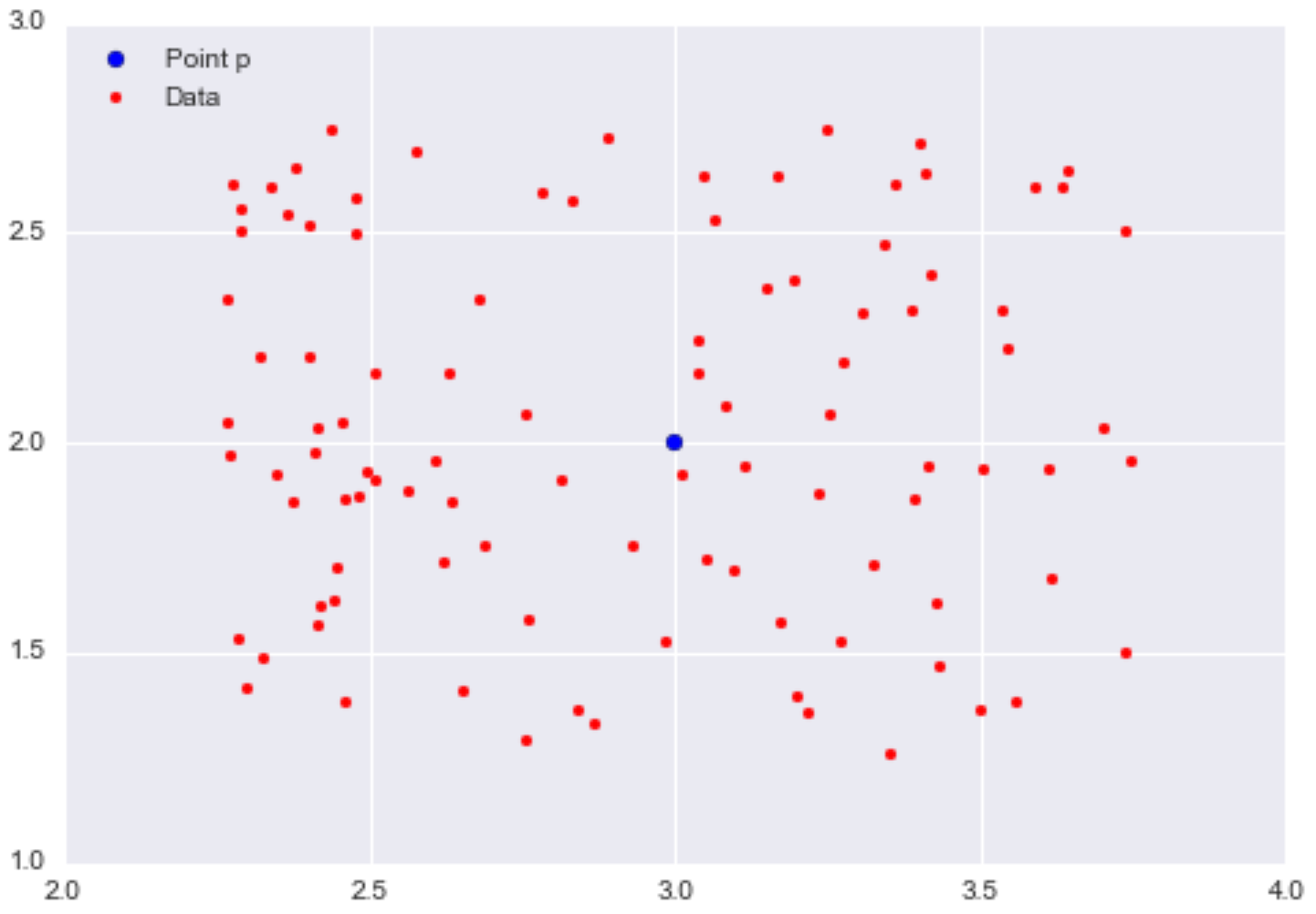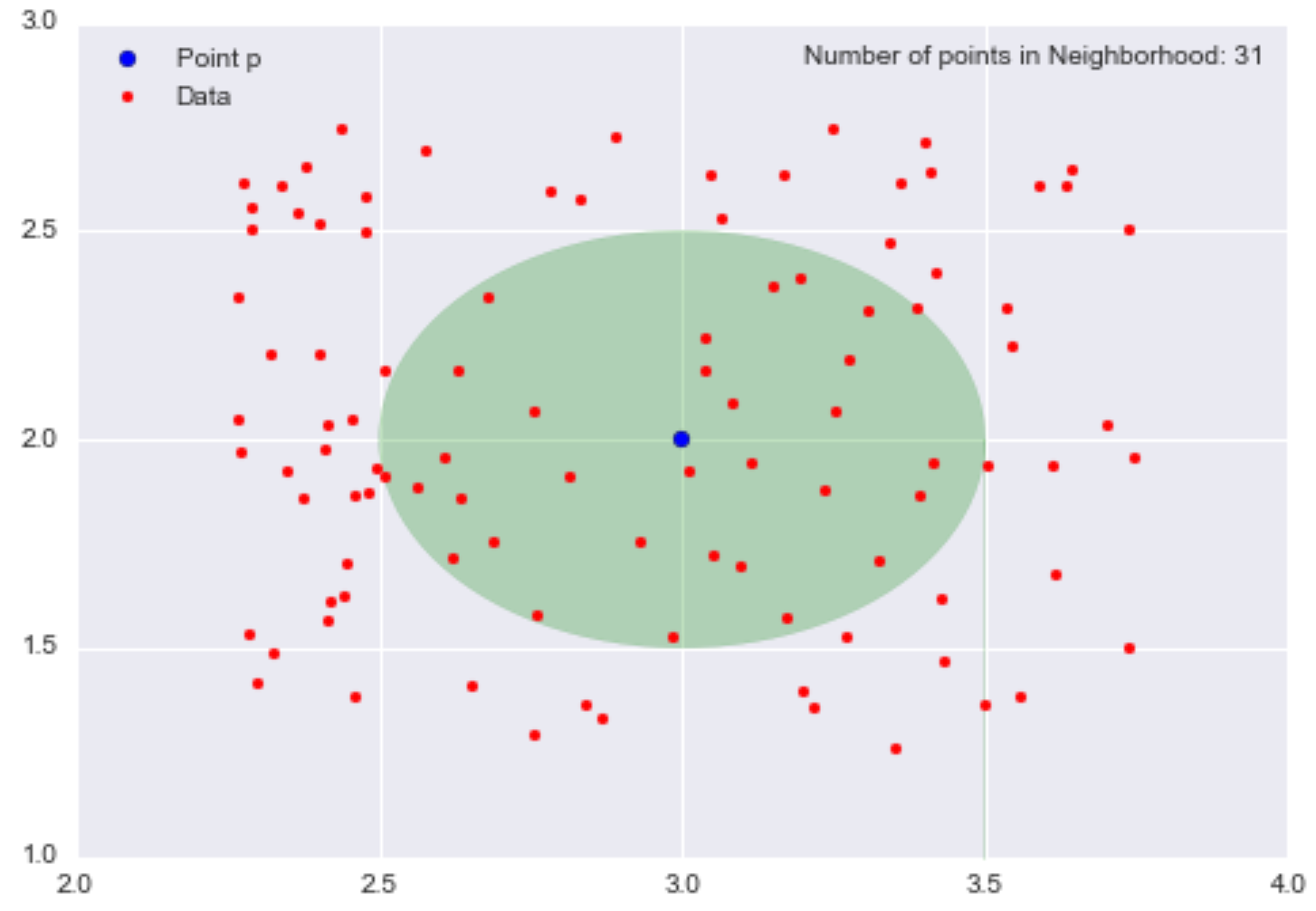In the previous posts of the ‘Clustering in ML’ Series, we looked at what clustering is and learned about Centroid-Based Clustering methods in ML. In this part, we will discuss another clustering method known as Density-Based Clustering.

In the previous post, we learned about k-means which is easy to understand and implement in practice. That algorithm has no notion of outliers, so all points are assigned to a cluster even if they do not belong in any. In the domain of anomaly detection, this causes problems as anomalous points will be assigned to the same cluster as “normal” data points. The anomalous points pull the cluster centroid towards them, making it harder to classify them as anomalous points. K-means perform best when clusters are:

• “round” or spherical
• equally sized
• equally dense
• most dense in the center of the sphere
• not contaminated by noise/outliers.

So when the datasets have clusters with arbitrary shapes, of different sizes, with different densities, or have some noise or outliers, Density-Based Clustering helps.

Density-Based Clustering refers to unsupervised learning methods that identify distinctive groups/clusters in the data, based on the idea that a cluster in data space is a contiguous region of high point density, separated from other such clusters by contiguous regions of low point density. The data points in the separating regions of low point density are typically considered noise.

### Intuition behind Density-Based Clustering

To understand different density-based clustering we first need to know some topics, and the first one is ɛ-neighborhoods.

For some real-valued ɛ > 0 and some point p, the ɛ-neighborhood of p is defined as the set of points that are at most distance ɛ away from p.

If you think back to geometry, the shape in which all points are equidistant from the center is the circle. In 2D space, the ɛ-neighborhood of a point p is the set of points contained in a circle of radius ɛ, centered at p. In 3D space, the ɛ-neighborhood is a sphere of radius ɛ, centered at p, and in higher dimensional space, the ɛ-neighborhood is just the N-sphere of radius ɛ, centered at p.

Let’s understand using an example, 100 data points in the interval Y – [1,3] and X – [2,4]. Let p be the point (3,2).

##Reference

First, let’s consider the neighborhood of p with radius 0.5 (ɛ = 0.5), the set of points that are distance 0.5 away from p.

##Reference

The opaque green oval represents our neighborhood, and there are 31 data points in this neighborhood. It means that approximately one-third of the data points are contained within the neighborhood of p with a radius of 0.5.

We have now defined what we mean by a “neighborhood”, now we’ll introduce the next important concept: the notion of a “density” for a neighborhood.

We know that density = mass/volume. We can define the mass of the neighborhood as the number of data points contained within the neighborhood, and the volume of the neighborhood is the volume of the resulting shape of the neighborhood.

So according to the example which we have taken above, the mass is the number of data points in the neighborhood, so mass = 31. The volume is the area of the circle, so volume = π0.25 = π/4. Therefore, local density at point p = (3,2) = 31/(π/4) = 124/π ~= 39.5.

This value is meaningless by itself, but if we calculate the local density approximation for all points in our dataset, we could cluster our points by saying that points that are nearby and have similar local density approximations belong in the same cluster. If we decrease the value of ɛ, we can construct smaller neighborhoods (less volume) that would also contain fewer data points. Ideally, we want to identify highly dense neighborhoods where most of the data points are contained in these neighborhoods, but the volume of each of these neighborhoods is relatively small.

We have understood the general intuition behind density-based clustering. Now, we will discuss the most important and common density-based clustering algorithm known as DBSCAN.

### DBSCAN

Density-Based Spatial Clustering of Applications with Noise (DBSCAN) is the most widely used density-based algorithm. DBSCAN estimates the density by counting the number of points in a fixed-radius neighborhood i.e ɛ and claim that two points are connected only if they lie within each other’s neighborhood.

Characterization of points

• A point is a core point if it has more than a specified number of points (MinPts) within ɛ These points belong in a dense region and are at the interior of a cluster.
• A border point has fewer than MinPts within ɛ but is in the neighborhood of a core point.
• A noise point is any point that is not a core point or a border point.

A point “p” is said to be density reachable from a point “q” if point “p” is within ε distance from point “q” and “q” has the sufficient number of points in its neighbors which are within distance ε.

#### Algorithm

Input: N objects to be clusters and global parameters ɛ and MinPts.

Output: Clusters of objects

1. Arbitrarily select a point p.
2. Retrieve all point density reachable from p wrt ɛ and MinPts.
3. If P is a core point a cluster is formed.
4. If P is a border point, then there is no point that is density reachable, and DBSCAN moves to the next point.
5. This process is continued until all the points are processed.

Reference

1. Does not require a prior specification of the number of clusters.
2. Able to identify noise data while clustering.
3. DBSCAN algorithm is able to find arbitrarily-sized and arbitrarily-shaped clusters.